• +91 9971497814
• info@interviewmaterial.com

# Chapter 10 Straight lines Ex-10.3 Interview Questions Answers

### Related Subjects

Question 1 :
Reduce the following equations into slope-intercept form and find their slopes and the y-intercepts.
(i) x + 7y = 0 (ii) 6x + 3y – 5 = 0 (iii) y = 0

(i) x + 7y = 0
Given:
The equation is x + 7y = 0
Slope – intercept form is represented in the form ‘y = mx + c’, where m is the slope and c is the y intercept
So, the above equation can be expressed as
y = -1/7x + 0
∴ The above equation is of the form y = mx + c, where m = -1/7 and c = 0.
(ii) 6x + 3y – 5 = 0
Given:
The equation is 6x + 3y – 5 = 0
Slope – intercept form is represented in the form ‘y = mx + c’, where m is the slope and c is the y intercept
So, the above equation can be expressed as
3y = -6x + 5
y = -6/3x + 5/3
= -2x + 5/3
∴ The above equation is of the form y = mx + c, where m = -2 and c = 5/3.
(iii) y = 0
Given:
The equation is y = 0
Slope – intercept form is given by ‘y = mx + c’, where m is the slope and c is the y intercept
y = 0 × x + 0
∴ The above equation is of the form y = mx + c, where m = 0 and c = 0.

Question 2 :
Reduce the following equations into intercept form and find their intercepts on the axes.
(i) 3x + 2y – 12 = 0
(ii) 4x – 3y = 6
(iii) 3y + 2 = 0

(i) 3x + 2y – 12 = 0
Given:
The equation is 3x + 2y – 12 = 0
Equation of line in intercept form is given by x/a + y/b = 1, where ‘a’ and ‘b’ are intercepts on x axis and y – axis respectively.
So, 3x + 2y = 12
now let us divide both sides by 12, we get
3x/12 + 2y/12 = 12/12
x/4 + y/6 = 1
∴ The above equation is of the form x/a + y/b = 1, where a = 4, b = 6
Intercept on x – axis is 4
Intercept on y – axis is 6
(ii) 4x – 3y = 6
Given:
The equation is 4x – 3y = 6
Equation of line in intercept form is given by x/a + y/b = 1, where ‘a’ and ‘b’ are intercepts on x axis and y – axis respectively.
So, 4x – 3y = 6
Now let us divide both sides by 6, we get
4x/6 – 3y/6 = 6/6
2x/3 – y/2 = 1
x/(3/2) + y/(-2) = 1
∴ The above equation is of the form x/a + y/b = 1, where a = 3/2, b = -2
Intercept on x – axis is 3/2
Intercept on y – axis is -2
(iii) 3y + 2 = 0
Given:
The equation is 3y + 2 = 0
Equation of line in intercept form is given by x/a + y/b = 1, where ‘a’ and ‘b’ are intercepts on x axis and y – axis respectively.
So, 3y = -2
Now, let us divide both sides by -2, we get
3y/-2 = -2/-2
3y/-2 = 1
y/(-2/3) = 1
∴ The above equation is of the form x/a + y/b = 1, where a = 0, b = -2/3
Intercept on x – axis is 0
Intercept on y – axis is -2/3

Question 3 :
Reduce the following equations into normal form. Find their perpendicular distances from the origin and angle between perpendicular and the positive x-axis.
(i) x – √3y + 8 = 0
(ii) y – 2 = 0
(iii) x – y = 4

(i) x – √3y + 8 = 0

Given:

The equation is x – √3y + 8 = 0

Equation of line in normal form is given by x cos θ + y sinθ = p where ‘θ’ is the angle between perpendicular and positive x axis and ‘p’is perpendicular distance from origin.

So now, x – √3y + 8 = 0

x – √3y = -8

Divide both the sides by √(12 + (√3)2)= √(1 + 3) = √4 = 2

x/2 – √3y/2 = -8/2

(-1/2)x + √3/2y = 4

This is in the form of: x cos 120o + y sin120o = 4

The above equation is of the form x cos θ + y sin θ = p,where θ = 120° and p = 4.

Perpendicular distance of line from origin = 4

Angle between perpendicular and positive x – axis = 120°

(ii) y – 2 = 0

Given:

The equation is y – 2 = 0

Equation of line in normal form is given by x cos θ + y sinθ = p where ‘θ’ is the angle between perpendicular and positive x axis and ‘p’is perpendicular distance from origin.

So now, 0 × x + 1 × y = 2

Divide both sides by √(02 + 12) =√1 = 1

0 (x) + 1 (y) = 2

This is in the form of: x cos 90o + y sin 90o =2

The above equation is of the form x cos θ + y sin θ = p,where θ = 90° and p = 2.

Perpendicular distance of line from origin = 2

Angle between perpendicular and positive x – axis = 90°

(iii) x – y = 4

Given:

The equation is x – y + 4 = 0

Equation of line in normal form is given by x cos θ + y sinθ = p where ‘θ’ is the angle between perpendicular and positive x axis and ‘p’is perpendicular distance from origin.

So now, x – y = 4

Divide both the sides by √(12 + 12)= √(1+1) = √2

x/√2 – y/√2 = 4/√2

(1/√2)x + (-1/√2)y = 2√2

This is in the form: x cos 315o + y sin 315o =2√2

The above equation is of the form x cos θ + y sin θ = p,where θ = 315° and p = 2√2.

Perpendicular distance of line from origin = 2√2

Angle between perpendicular and positive x – axis = 315°

Question 4 : Find the distance of the point (–1, 1) from the line 12(x + 6) = 5(y – 2).

Given:
The equation of the line is 12(x + 6) = 5(y – 2).
12x + 72 = 5y – 10
12x – 5y + 82 = 0 … (1)
Now, compare equation (1) with general equation of line Ax + By + C = 0, where A = 12, B = –5, and C = 82
Perpendicular distance (d) of a line Ax + By + C = 0 from a point (x1, y1) is given by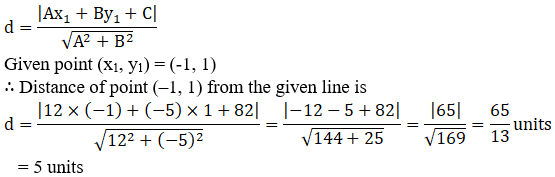∴ The distance is 5 units.

Question 5 : Find the points on the x-axis, whose distances from the line x/3 + y/4 = 1 are 4 units.

Given:
The equation of line is x/3 + y/4 = 1
4x + 3y = 12
4x + 3y – 12 = 0 …. (1)
Now, compare equation (1) with general equation of line Ax + By + C = 0, where A = 4, B = 3, and C = -12
Let (a, 0) be the point on the x-axis, whose distance from the given line is 4 units.
So, the perpendicular distance (d) of a line Ax + By + C = 0 from a point (x1, y1) is given by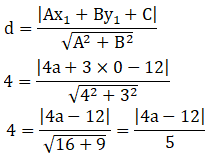|4a – 12| = 4 × 5
± (4a – 12) = 20
4a – 12 = 20 or – (4a – 12) = 20
4a = 20 + 12 or 4a = -20 + 12
a = 32/4 or a = -8/4
a = 8 or a = -2
∴ The required points on the x – axis are (-2, 0) and (8, 0)

Question 6 :
Find the distance between parallel lines
(i) 15x + 8y – 34 = 0 and 15x + 8y + 31 = 0
(ii) l(x + y) + p = 0 and l (x + y) – r = 0

(i) 15x + 8y – 34 = 0 and 15x + 8y + 31 = 0

Given:

The parallel lines are 15x + 8y – 34 = 0 and 15x + 8y + 31 =0.

By using the formula,

The distance (d) between parallel lines Ax + By + C1 =0 and Ax + By + C2 = 0 is given by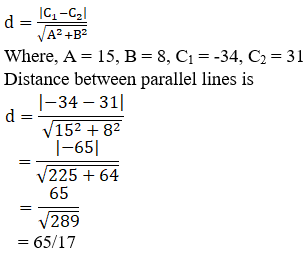The distance between parallel lines is 65/17

(ii) l(x + y) + p = 0 and l (x + y) – r = 0

Given:

The parallel lines are l (x + y) + p = 0 and l (x + y) – r =0.

lx + ly + p = 0 and lx + ly – r = 0

by using the formula,

The distance (d) between parallel lines Ax + By + C1 =0 and Ax + By + C2 = 0 is given by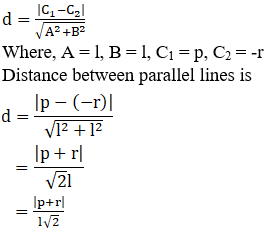The distance between parallel lines is |p+r|/l√2

Question 7 : Find equation of the line parallel to the line 3x − 4y + 2 = 0 and passing through the point (–2, 3).

Given:

The line is 3x – 4y + 2 = 0

So, y = 3x/4 + 2/4

= 3x/4 + ½

Which is of the form y = mx + c, where m is the slope of thegiven line.

The slope of the given line is 3/4

We know that parallel line have same slope.

Slope of other line = m = 3/4

Equation of line having slope m and passing through (x1,y1) is given by

y – y1 = m (x – x1)

Equation of line having slope 3/4 and passing through(-2, 3) is

y – 3 = ¾ (x – (-2))

4y – 3 × 4 = 3x + 3 × 2

3x – 4y = 18

The equation is 3x – 4y = 18

Question 8 : Find equation of the line perpendicular to the line x – 7y + 5 = 0 and having x intercept 3.

Given:

The equation of line is x – 7y + 5 = 0

So, y = 1/7x + 5/7 [which is of the form y = mx + c, where mis the slope of the given line.]

Slope of the given line is 1/7

Slope of the line perpendicular to the line having slope mis -1/m

Slope of the line perpendicular to the line having a slopeof 1/7 is -1/(1/7) = -7

So, the equation of line with slope -7 and x intercept 3 isgiven by y = m(x – d)

y = -7 (x – 3)

y = -7x + 21

7x + y = 21

The equation is 7x + y = 21

Question 9 : Find angles between the lines √3x + y = 1 and x + √3y = 1.

Given:
The lines are √3x + y = 1 and x + √3y = 1
So, y = -√3x + 1 … (1) and
y = -1/√3x + 1/√3 …. (2)
Slope of line (1) is m1 = -√3, while the slope of line (2) is m2 = -1/√3
Let θ be the angle between two lines
So,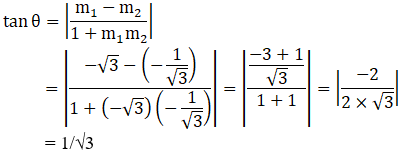θ = 30°
∴ The angle between the given lines is either 30° or 180°- 30° = 150°

Question 10 : The line through the points (h, 3) and (4, 1) intersects the line 7x − 9y −19 = 0. At right angle. Find the value of h.

Let the slope of the line passing through (h, 3) and (4, 1) be m1
Then, m1 = (1-3)/(4-h) = -2/(4-h)
Let the slope of line 7x – 9y – 19 = 0 be m2
7x – 9y – 19 = 0
So, y = 7/9x – 19/9
m2 = 7/9
Since, the given lines are perpendicular
m1 × m2 = -1
-2/(4-h) × 7/9 = -1
-14/(36-9h) = -1
-14 = -1 × (36 – 9h)
36 – 9h = 14
9h = 36 – 14
h = 22/9
∴ The value of h is 22/9

Todays Deals### Chapter 10 Straight lines Ex-10.3 Contributorskrishan

Name:
Email:

# Latest News# 9000 interview questions in different categories